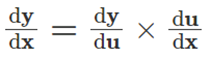top of page
Search

# All you want to know about DIFFERENTIATION:

The concept of differentiation is one of the two most important concepts in calculus, along with integration. Calculating a function's derivative is called differentiation. Using one of a function's variables, differentiation determines the instantaneous rate at which it changes.

One of the most common examples is the change in displacement over time, known as velocity. A derivative cannot be found if an anti-differentiation is performed.

If x is a variable and y is another variable, then dy/dx represents the rate of change of x with respect to y. The derivative of a function can be expressed as f'(x) = dy/dx, where y = f(x) is any function.

###### In mathematics, what is differentiation?

Differentiation is defined in mathematics as the derivative of a function based on an independent variable. An independent variable can be changed by one unit by using differentiation in calculus.

Suppose y = f(x) is a function of x. In this case, the rate of change of "y" per unit change in "x" is as follows: dy / dx

Near any point 'x’ if f(x) undergoes a small change in 'h,' then the derivative isFunctions as limits of derivatives:

If x belongs to the domain of definition of a real-valued function (f), then we can predict the derivative by:

f'(a) = limh→0[f(x + h) – f(x)]/h

provided this limit exists.

Let us see an example here for a better understanding.

Example: Find the derivative of f(x) = 2x, at x =3.

Solution:

By using the above formulas, we can find,

f'(3) = limh→0 [f(3 + h) – f(3)]/h = limh→0[2(3 + h) – 2(3)]/h

f'(3) = limh→0 [6 + 2h – 6]/h

f'(3) = limh→0 2h/h

f'(3) = limh→0 2 = 2

## Notations:

When a function is denoted as y = f(x), the derivative is indicated by the following notations.

1. D(y) or D[f(x)] is called Euler’s notation.

2. dy/dx is called Leibniz’s notation.

3. F’(x) is called Lagrange’s notation.

In mathematics, differentiation refers to determining an object's derivative at any point in time.

## Linear and Non-Linear Functions:

Functions are generally classified into two categories under Calculus, namely:

(i) Linear functions

(ii) Non-linear function

Through its domain, a linear function varies at a constant rate. As a result, the overall rate of change of a function is the same as the rate of change at any particular point.

In the case of non-linear functions, the rate of change varies from point to point. The variation depends on the nature of the function.

A derivative of a function is the rate of change of that function at a given point.

## Differentiation Formulas:

The important Differentiation formulas are given below in the table. Here, let us consider f(x) as a function, and f'(x) is the derivative of the function.

· If f(x) = tan (x), then f'(x) = sec2x

· If f(x) = cos (x), then f'(x) = -sin x

· If f(x) = sin (x), then f'(x) = cos x

· If f(x) = ln(x), then f'(x) = 1/x

· If f(x) = ex, then f'(x) = ex

· If f(x) = xn, where n is any fraction or integer, then f'(x) = nxn-1

· If f(x) = k, where k is a constant, then f'(x) = 0

## Rules of Differentiation:

Following are the basic differentiation rules:

• Sum and Difference Rule

• Product Rule

• Quotient Rule

• Chain Rule

Here are all the rules we need to discuss.

## Rule of Sum or Difference:

If a function is the sum or difference of two functions, the derivative of the function is the sum or difference of the individual functions.

If f(x) = u(x) ± v(x)

then, f'(x) = u'(x) ± v'(x)

## Product Rule:

As per the product rule, if the function f(x) is product of two functions u(x) and v(x), the derivative of the function is,

IfQuotient rule:

If the function f(x) is in the form of two functions [u(x)]/[v(x)], the derivative of the function is

If,Chain Rule:

If a function y = f(x) = g(u) and if u = h(x), then the chain rule for differentiation is defined as,It plays an important role in the substitution method, which helps to perform the differentiation of complex functions

Practical application of differentiation:

Differentiation can be used to find the rate of change of one quantity with respect to another quantity. Some of the examples are:

• acceleration: rate of change of velocity over time

• Differential functions are used to calculate the highest and lowest points of a curve on a graph and to find its turning points

• find tangents and normal of curves

## Solved Examples:

Q.1: Differentiate f(x) = 6x3 – 9x + 4 with respect to x. Solution: Given: f(x) = 6x3 – 9x + 4

On differentiating both the sides w.r.t x, we get;

f'(x) = (3)(6)x2 – 9

f'(x) = 18x2 – 9

Some Sample Lectures on DIFFERENTIATION by Y.S Sir: